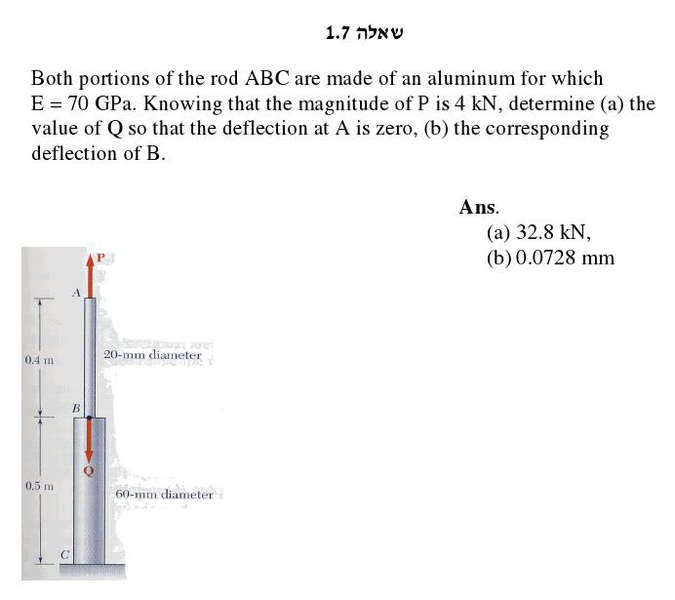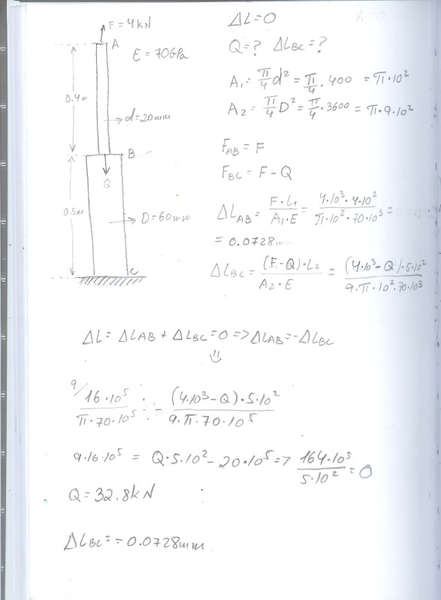# Mechanics of Materials - Where's my mistake?

• Femme_physics
The total expansion is indeed 0.425 mm.That is at point A!So at point A you should have an expansion of 0.425 mm.However, at point B the part of the bar to the right will be expanded less, by as much as ΔL_AB.Same thing at point C, where the expansion is only ΔL_CD.Finally at point D, the expansion would have to be zero.Connect the dots with lines and you have your movement...graph. :)

Gold Member

## Homework Statement

http://img85.imageshack.us/img85/6346/missrd.jpg [Broken]

Across the axis of a steel rod act 3 forces:

F1 = 80 kN
F2 = 50 kN
F3 = 30 kN

Crossection area of rod = 4cm2

What are the forces that are being made a the different parts of the rod, and what will be it's overall lengthening? Describe the course of the axial forces and the stresses through the rod.. measurements are given in cm.

E = 2 x 105

(the answers are in the screenshot above)

## Homework Equations

[PLAIN]http://img831.imageshack.us/img831/533/neededformulas.jpg[b] [Broken]

3. The Attempt at a Solution [/b]

http://img840.imageshack.us/img840/6550/solutionto.jpg [Broken]

From some reason I'm getting different answers than given...but why?

Last edited by a moderator:
Suppose you would saw the steel rod in half somewhere between A and B.
What would have to be the force at that point to maintain equilibrium?

Hint: it is not 20 kN, which is what you calculated for FAB.

Suppose you would saw the steel rod in half somewhere between A and B.
What would have to be the force at that point to maintain equilibrium?
It's not 20 [kN]? Then what it is? I thought you just do F3 - F2, no?

Pick a point between A and B and sum all the forces to the left.

Or equivalently sum all the forces to the right.

Good! And yes, the stress force between A and B is 30 kN.

If you divide it by the surface, do you get a number that looks like the answer key?

ThanksI'll try to fix it all at home tonight and re-scan it. Got it. You're the best. I'll go hunt some op-amps nowLast edited by a moderator:
It matters if you sum the forces to the left or the forces to the right.
The difference is a minus sign.
In your case F_CD has an inconsistent sign.
It's probably best to consistently pick either the one or the other.

Furthermore you will want your stress force to be positive, if it expands the bar.
On the section AB the only real operative force is F3 which points to the left, which would expand the bar.
So you will want F_AB to be positive and have the other forces match sign (by summing on the same side).

It matters if you sum the forces to the left or the forces to the right.
The difference is a minus sign.
In your case F_CD has an inconsistent sign.
It's probably best to consistently pick either the one or the other.

Furthermore you will want your stress force to be positive, if it expands the bar.
On the section AB the only real operative force is F3 which points to the left, which would expand the bar.
So you will want F_AB to be positive and have the other forces match sign (by summing on the same side).

Ah, I got itI UNDERSTAND FULLY. Thank you.
http://img585.imageshack.us/img585/3945/f1000.jpg [Broken]

There's just one thing...drawing a "Movement graph of every point". I'm not sure how to do those. Can you help with me with it?

Last edited by a moderator:
Femme_physics said:
Ah, I got itI UNDERSTAND FULLY. Thank you.

Good.

Femme_physics said:
There's just one thing...drawing a "Movement graph of every point". I'm not sure how to do those. Can you help with me with it?

Sure.
I see you already drew force and tension diagrams... but the numbers don't match your diagrams...

As for the "movement graph", I take it that would be the graph indicating the expansion of the bar at each point.
How much is the total expansion at each of the points C, B, and A (counting from the wall)?

Sure.
I see you already drew force and tension diagrams... but the numbers don't match your diagrams...

Ah, forgot to change the figures, oopsie!

As for the "movement graph", I take it that would be the graph indicating the expansion of the bar at each point.
How much is the total expansion at each of the points C, B, and A (counting from the wall)?

Haven't we calculated it? 0.425 mm according to my last scan.

So this is what I got...

http://img444.imageshack.us/img444/6533/111jo.jpg [Broken]

Last edited by a moderator:
I see you got the proper force and tension diagrams now. :)

As for the movement diagram.
The total expansion is indeed 0.425 mm.
That is at point A!
So at point A you should have an expansion of 0.425 mm.
However, at point B the part of the bar to the right will be expanded less, by as much as ΔL_AB.
Same thing at point C, where the expansion is only ΔL_CD.
Finally at point D, the expansion would have to be zero.

Connect the dots with lines and you have your movement diagram!

That is at point A!
So at point A you should have an expansion of 0.425 mm.
However, at point B the part of the bar to the right will be expanded less, by as much as ΔL_AB.
Same thing at point C, where the expansion is only ΔL_CD.
Finally at point D, the expansion would have to be zero.

Femme_physics said:

$$\begin{array}{| l | l |} \hline \\ \textrm{At }D & ΔL = 0 \\ \textrm{At }C & ΔL = ΔL_{CD} \\ \textrm{At }B & ΔL = ΔL_{CD} + ΔL_{BC} \\ \textrm{At }A & ΔL = ΔL_{CD} + ΔL_{BC} + ΔL_{AB} \\ \end{array}$$

Last edited by a moderator:
Gotcha!2 questions

1) I noticed I converted 4cm^2 to 400mm^2... shouldn't it have been 40mm^2?

2) For doing that last graph, does 0 alway start from the wall?

Femme_physics said:
2 questions

1) I noticed I converted 4cm^2 to 400mm^2... shouldn't it have been 40mm^2?

No.

I recommend converting units like this:

4 cm^2 = 4 (1 cm)^2 = 4 (10 mm)^2 = 4 (10)^2 (1 mm)^2 = 400 mm^2

That is, put the unit between parentheses, and replace it by the other unit, including the conversion-factor.
Then work out any powers and such.

Femme_physics said:
2) For doing that last graph, does 0 alway start from the wall?

Yes.

For length expansions, at the wall where it is fixed, there is zero length that could be expanded, so the expansion is zero.

Yes.

For length expansions, at the wall where it is fixed, there is zero length that could be expanded, so the expansion is zero.

Thanks.

No.

I recommend converting units like this:

4 cm^2 = 4 (1 cm)^2 = 4 (10 mm)^2 = 4 (10)^2 (1 mm)^2 = 400 mm^2

That is, put the unit between parentheses, and replace it by the other unit, including the conversion-factor.
Then work out any powers and such.

I'm a bit confused how you made this conversation. All I know is that

1m = 100cm = 1000mm

Therefor 4cm = 40mm
and 40cm = 400mm

So how does the fact it's squared changes things?

Hello,
another way to look at it for the conversion is
$$4 cm^2=4(1 cm)(1 cm)=4(10 mm)(10 mm)=400 mm^2$$

So

1m2 = 1 (1000mm) (1000mm) = 1000000mm2

?

Correct.

Got it. ThanksAherm, I don't while I got you here (and I don't really want to start a new thread), I have the full solution of this question

http://img100.imageshack.us/img100/1631/prrobbb.jpg [Broken]

where I have to find stresses through the rod and total distortion

But I appear to be one decimal point wrong. Can you find it? My friend actually solved it and I confirmed her solution, I even resolved it on another paper and got the same result. Are we wrong or are the teacher answers wrong?

here:

http://img689.imageshack.us/img689/8797/hersol1000.jpg [Broken]

Last edited by a moderator:

Aha, I knew itThank Klaas, this helps confirm and I will show it to my teacher on Friday.

So, me and her set on yet another exercise and we got different results. We didn't make a bet this time...(she probably wants to, I'm unsure if I used the correct formula for area). The only problem is that this time my results are different than hers. So we got 3 different sets of results. My teacher's, mine, and her's. We would really appreciate your expert's opinion on this one (even delayed the control engineering exercise for this). Who do you think got it?

The problem:

http://img97.imageshack.us/img97/1213/exdia.jpg [Broken]

Solution one (hers):

http://img825.imageshack.us/img825/5094/darsol.jpg [Broken]

Solution two (mine):

http://img59.imageshack.us/img59/1221/orsolwhatswrong.jpg [Broken]

Last edited by a moderator:
You both seem to have trouble with the calculation of area.

Your friend seems to have calculated the surface area of the inside of the rod, but that is empty air.

You seem to have treated the thickness of the wall as if it is a diameter on its own, which it isn't.

At top view of the rod would show 2 concentric circles.
The cross sectional area of the rod is the surface of the outer circle minus the surface of the inner circle.

I just calculated and u were right. Actually, I was rushing in with the area formula and I knew that was the problem. I should've just thought a bit and did it the long way instead of looking for shortcuts. Thank you very much ILS, you're the best.

Hi friend of Fp!I only got one minor comment.
Your graph of the length expansions has its zero at a.
But the zero should be at c, which is where the ground is, and where there will be no contraction.

Hi I am the friend, just to confirm:

A= π/4*(D)^2-π/4*(D-2α)^2

niece of md. said:
Hi I am the friend, just to confirm:

A= π/4*(D)^2-π/4*(D-2α)^2

Yep. That is the proper formula for the area of the cross section.

Thanks I'll change that, but I am allowed to make those mistakes since I don't really study it, I just do it for fun :)

Well, I'm just doing this for fun too.
But I don't allow myself many mistakes! ;)

Well, I do it for fun and for a degree! Thanks for helping out ILSwe got it done, and are off to solve more complicated stuff now. Hopefully it'll go more smoothly.

I was trying to solve this exercise but since it's in English I wasn't sure I understood it.this is what I did:I was a little confused with +/- sings on F(bc).